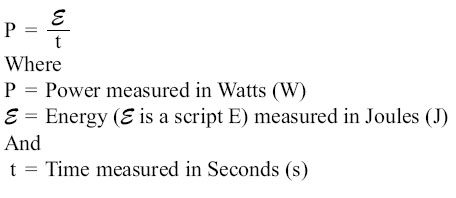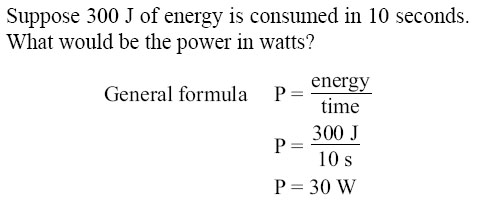Rectangular Conductors (Bus Bars) To compute the cross-sectional area of a conductor in square mils, the length in mils of one side is squared. In the case of a rectangular conductor, the length of one side is multiplied by the length of the other. For example, a common rectangular bus bar (large, special conductor) is 3/8 inch thick and 4 inches wide. The 3/8-inch thickness may be expressed as 0.375 inch. Since 1,000 mils equal 1 inch, the width in inches can be converted to 4,000 mils. The cross-sectional area of the rectangular conductor is found by converting 0.375 to mils (375 mils × 4,000 mils = 1,500,000 square mils). Power and Energy Power in an Electrical Circuit This section covers power in the DC circuit and energy consumption. Whether referring to mechanical or electrical systems, power is defined as the rate of energy consumption or conversion within that system — that is, the amount of energy used or converted in a given amount of time. From the scientific discipline of physics, the fundamental expression for power is:The unit measurement for power is the watt (W), which refers to a rate of energy conversion of 1 joule/second. Therefore, the number of joules consumed in 1 second is equal to the number of watts. A simple example is given below.The watt is named for James Watt, the inventor of the steam engine. Watt devised an experiment to measure the power of a horse in order to find a means of measuring the mechanical power of his steam engine. One horsepower is required to move 33,000 pounds 1 foot in 1 minute. Since power is the rate of doing work, it is equivalent to the work divided by time. Stated as a formula, this is: Power=33,000 ft-lb/60 secP = 550 ft-lb/sec Electrical power can be rated in a similar manner. For example, an electric motor rated as a 1 horsepower motor requires 746 watts of electrical energy.
 ©AvStop Online Magazine                                                                                                                                                      Contact Us              Return To Books